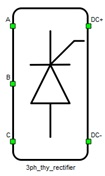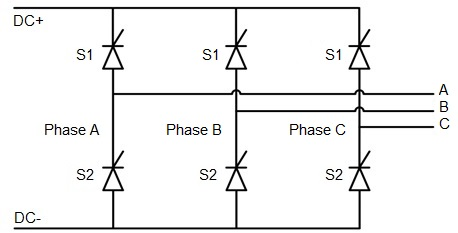# Three Phase Thyristor Rectifier

Description of the Three Phase Thyristor Rectifier component in Schematic Editor

A block diagram and input parameters for a single three-phase thyristor rectifier are given in Table 1.

Table 1. A three-phase thyristor rectifier component in the Schematic Editor core library
component component dialog window component parametersThree phase thyristor rectifier• General
• Control source (Digital inputs/ Model)
• Digital input pins for:
• Phase A S1 (1..32)
• Phase A S2 (1..32)
• Phase B S1 (1..32)
• Phase B S2 (1..32)
• Phase C S1 (1..32)
• Phase C S2 (1..32)
• gate_logic (active high, active low)
• _control_property (all high, all low)
• Gate control enabling (enables usage of external digital signal to enable switching)
• Sen (digital input selection for gate enable)
• Sen_logic (active high, active low)
• PESB Optimization
Weight = 3

Digital inputs, when selected as the control input option, enables assigning gate drive inputs to any of the digital input pins (from 1 to 32/64). For example, if Phase A S1 is assigned to 1, the digital input pin 1 is routed to the Phase A S1 switch gate drive. In addition, the gate_logic parameter selects either active high (High-level input voltage VIH turns on the switch), or active low (Low-level input voltage VIL turns on the switch) gate drive logic, depending on the external controller design being tested.

Model control, when selected for the Control parameter, enables setting the thyristor gate drive signals directly from signal processing model. The input pin that appear on the top of the rectifier requires a vector input of six gate drive signals in the following order: [Phase A S1, Phase A S2, Phase B S1, Phase B S2, Phase C S1, Phase C S2]. When controlled from the model, logic is always active high.

PESB Optimization, optimizes the switching block to reduce the memory requirements of the model.

A schematic block diagram of a three-phase thyristor rectifier block is given in Figure 1.Figure 1. A schematic block diagram of a three-phase thyristor rectifier block diagram with corresponding switch naming

## Losses calculation

When the Losses calculation property is enabled, the component will calculate switching and conduction power losses for all switching elements (Thyristors). Switching power losses are calculated as a function of current, voltage, and temperature using 3D Look-up tables (LUTs). Also, 2D input for losses is supported. When a 2D losses table is inserted, it assumes only current and temperature dependance. Conduction power losses can be defined as a function of current and temperature using Vt Look-up tables. These LUTs can be either 1D or 2D. If the LUT is a 1D table, the forward voltage drop depends only on current. If the LUT is a 2D table, the forward voltage drop dependence on the junction temperature is included.

Input/output power losses ports receive/generate vectors of six elements. The first element (index 0) is the upper thyristor in phase A, the second element (index 1) is the bottom thyristor in phase A. The next two elements are the phase B upper and bottom thyristor, respectively. The 4th and 5th elements follow this same pattern for phase C. Available mask properties are:
• Current values - Switching elements current axis [A]
• Voltage values - Switching elements voltage axis [V]
• Temp values - Switching elements temperature axis [°C]
• Vt table - Thyristor forward voltage drop, f(I,T) [V]
• Et on table - Thyristor switching ON losses, output energy, f(I, V, T) [J]

## Temperatures calculation

When the Temperatures calculation property is enabled, the component calculates the combined power losses (P_loss) and junction temperatures (T_junctions) for all switching elements (Thyristors). Combined power losses represent the sum of the calculated switching and conduction losses transfered through an internally generated Thermal network component. The internally generated Thermal network component also calculates the junction temperatures from power losses, input case temperatures, and provided thermal model parameters. Input/output ports for temperature calculation are vectors of six elements and they are indexed in the same way as explained in the Losses calculation section. Additional temperature calculation mask properties include:
• Thermal networks type - Defines type of internal thermal network
• Thyristor Rth - List of thermal resistances for thyristor
• Thyristor Tth / Cth- List of thermal time constants or thermal capacitances for thyristor
• Calculation execution rate - Execution rate in [s] for the losses and temperature calculation logic

## Digital Alias

If a converter is controlled by digital inputs, an alias for every digital input used by the converter will be created. Digital input aliases will be available under the Digital inputs list alongside existing Digital input signals. The alias will be shown as Converter_name.Switch_name, where Converter_name is name of the converter component and Switch_name is name of the controllable switch in the converter.